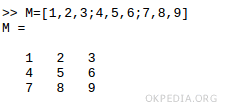# How to reverse the order of rows of a matrix in Matlab Octave

To flip a matrix right left in Matlab and Octave use the function fliplr().

fliplr(M)

Example

Create a square matrix M with three rows and three columns.Flip the matrix right to left with the function fliplr(M).The output result is the matrix 3x3 with its columns flipped in the left-right direction

https://how.okpedia.org/en/matlab/how-to-reverse-the-order-of-rows-of-a-matrix-in-matlab-octaveReport us an error or send a suggestion to improve this page

Matrix in Matlab/Octave